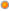## PMAT 32622: Mathematical Methods

User Rating: 5 / 5Course Code            : PMAT 32622

Title                          : Mathematical Methods

Pre-requisites          : PMAT 22583

Learning Outcomes:

At the end of this course, the student should be able to solve different types ordinary and partial differential equations using various techniques learned.

Course Contents:

Special Functions: Legendre Polynomials, Bessel Functions

Laplace Transforms: Laplace Transform. Linearity, Shifting Theorems, Transforms of Derivatives and Integrals, Unit Step Function (Heaviside Function), Differentiation and Integration of Transforms.

Fourier Series: Fourier Series, Even and Odd Functions, Half-Range Expansions, Fourier Integral, Fourier Cosine and Sine Transforms

Partial Differential Equations (PDEs): Basic Concepts of PDEs, Wave Equation

Solution by Separating Variables, Use of Fourier Series, D’Alembert’s Solution of the Wave Equation. Characteristics

Method of Teaching and Learning: A combination of lectures and tutorial discussions.

Assessment: Based on tutorials, tests and end of course examination.